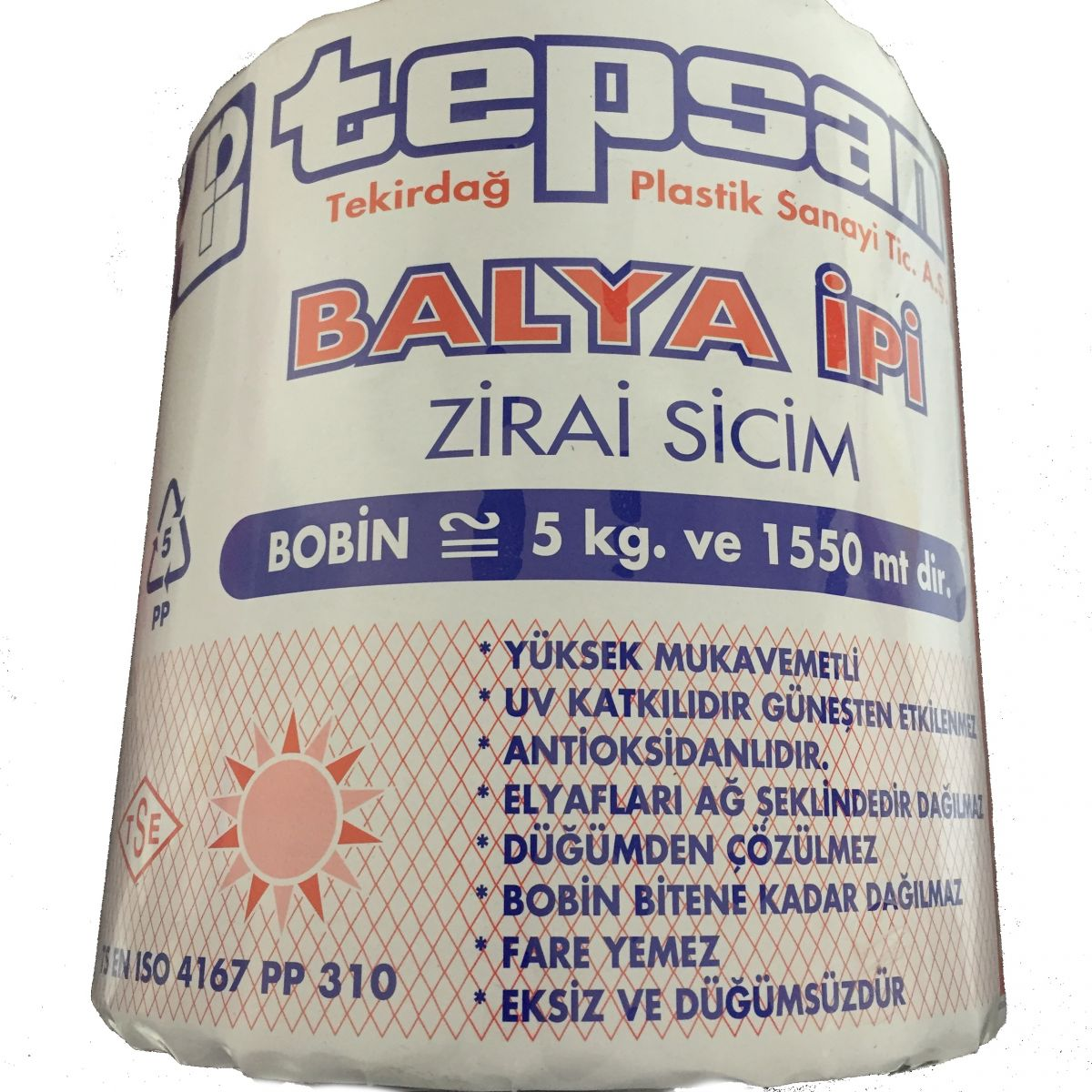# TECHNICAL TIPS FOR BALER TWINE SELECTIONLet's find answers to many unknown questions about the Baler Twine. The most correct way to get rid of invisible costs is to pay attention to some technical features and to make an accurate calculation.

Here are some important topics:

How many meters of twine do you get in a spool? How to calculate?

1. Weigh the spool you bought first. As an example, let's proceed as follows. As Tepsan, our baler twines are sold in 5 kg spools.

2. Cut a 10 meter long section from the coil and then weigh it. You will find approximately 32,258 gr in Tepsan Baler Twine.

3. If you divide the result by 10, you will find out how many grams of 1 meter of twine come. The result will be 3,2258 in the Tepsan Baler Twine.

4. In the next step, divide 1000 g (1 kg) by the result. Thus, you can find out how many meters of twine are in 1 kg. It will be approximately 310 meters in Tepsan. This is also a reference to the T310 number written on the Tepsan Baler Twine packaging.

5. If you multiply the figure you find by the weight of the spool (5 kg), you can find out how many meters of baler twine you take with 1 spool. In Tepsan Baler Twine spools you will find the result as 1550 meters.

## What does the breaking force mean? (Tensile)

It refers to the maximum stress of the string applied or the stress before it breaks. However, when it comes to the knot thrown by the machine, this figure has no significance. Because the knot is the weakest point of the twine.

The main issue here is not which baler twine used, but the shape of the knot of the baler that it throws for the bale.

## Node shapes of the baler

There are only two types of knotting on all balers in the world.

The first is the DEERING node, referred to as Standard, and the other is the CORMICK or LOOP knot.

There is a 70% break strength difference between the Standard knot made with the same twine and the Loop knot. The loop knot shows high performance in this regard.

Because of all this, baler twine cannot be selected only on the breaking strength figures. However, you can discuss this with your machine tool builder and request a change of knot shape.

## Why is the thickness and twist count of the baler twine important?

The thicker the twine is, the better its logic is not a measure in terms of use in baler twine and balers, it is even a great risk. As the twine thickens, short ends are formed during the knot and cause easily unraveling. In addition, as the number of twists in the twine increases, the risk of entanglement in the knotting mechanism of the bale string decreases. A loose baler twine can seriously damage your machine.